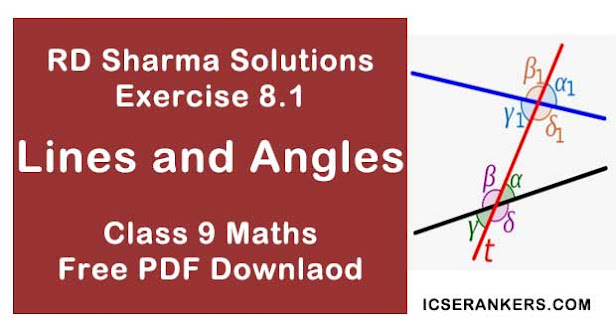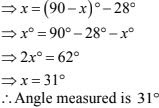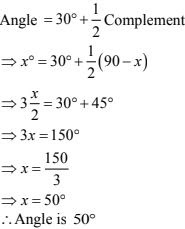# Chapter 8 Lines and Angles RD Sharma Solutions Exercise 8.1 Class 9 MathsChapter Name RD Sharma Chapter 8 Lines and Angles Exercise 8.1 Book Name RD Sharma Mathematics for Class 10 Other Exercises Exercise 8.2Exercise 8.3Exercise 8.4 Related Study NCERT Solutions for Class 10 Maths

### Exercise 8.1 Solutions

1. Write the complement of each of the following angles:
(i) 20°

(ii) 35°

(iii) 90°

(iv) 77°

(v) 30°

Solution

(i) Given angle is 20°
Since, the sum of an angle and its complement is 90°.
∴ its, complement will be  (90 - 20 = 70°)

(ii) Given angle is  35°
Since, the sum of an angle and its complement is 90°.
∴ its, complements will be (90 - 35° = 55°)

(iii) The given angle is 90°
Since, the sum of an angle and its complement is 90°.
∴ [its, complement will be (90 - 90° = 0°)]

(iv) The given angle is 77°
Since, the sum of an angle and its complement is 90°.
∴ its, complement will be (90 - 77° = 13°)

(v) The given angle is 30° .
Since, the sum of an angle and its complement is 90° .
∴ its, complement will be (90 - 30° = 60°)

2. Write the supplement of each of the following angles:
(i) 54°

(ii) 132°

(iii) 138°

Solution

(i) The given angle is 54°
Since, the sum of an angle and its supplement is 180°  .
∴  its, supplement will be 180° - 54° = 126°.

(ii) The given angle is  132°
Since, the sum of an angle and its supplement is 180° .
∴ its, supplement will be 180° - 132° = 48°

(iii) The given angle is 138°
Since, the sum of an angle and its supplement is 180° .
∴ its, supplement will be 180° - 138° = 42°

3. If an angle is 28° less than its complement, find its measure.

Solution

Angle measured will be 'x' say
∴ its complement will be (90 - x)°
It is given that
Angle = Complement - 28°4. If an angle is 30° more than one half of its complement, find the measure of the angle.
Solution
Angle measured will be 'x' say.
∴ its complement will be (90 - x)°
It is given that5. Two supplementary angles are in the ratio 4 : 5. Find the angles.
Solution
Supplementary angles are in the ratio 4 : 5
Let the angles be 4x and 5x
It is given that they are supplementary angles
∴ 4x + 5x = 180° x
⇒ 9x = 180°
⇒ x = 20°
Hence, 4x = 4(20) = 80°
5(x) = 5(20) = 100°
∴ Angles are 80° and 100°.

6. Two supplementary angles differ by 48°. Find the angles.
Solution
Given that two supplementary angles are differ by 48°
Let the angle measured is x°
∴ Its supplementary angle will be (180 - x)°
It is given that
(180 - x) - x = 98°
⇒ 180 - 48° = 2x
⇒ 132 = 2x
⇒ x = 132/2
⇒ x = 66°
Hence, 180 - x =114°
Therefore, angles are 66° and 114°.

7. An angle is equal to 8 times its complement. Determine its measure.
Solution
It is given that angle = 8 times its complement
Let 'x' be measured angle
⇒ angle = 8 complements
⇒ angle = 8(90° - x)  [∵ complement = (90° - x)]
⇒ x° = 8(90) - 8x
⇒ 9x = 720
⇒ x = 720/9 = 80
∴ The measured angle is 80°.

8. If the angles (2x - 10)° and (x - 5)° are complementary angles, find x.
Solution
Given that ,
(2x - 10)° and (x - 5)° are complementary angles.
Let x be the measured angle.
Since the angles are complementary
∴ Their sum will be 90°
⇒ (2x - 10) + (x - 5) = 90°
⇒ 3x - 15 = 90°
⇒ 3x = 90° + 15°
⇒ x = 105°/3 = 35°
⇒ x = 35°

9. If the complement of an angle is equal to the supplement of the thrice of it. Find the measure of the angle.
Solution
The angle measured will be 'x' say.
Its complementary angle is (90° - x) and
Its supplementary angle is (180° - 3x)
Given that,
Supplementary of thrice of the angle = (180° - 3x)
According to the given information
(90° - x) = (180° - 3x)
⇒ 3x - x = 180° - 90°
⇒ 2x = 90°
⇒  x = 45°
The angle measured is 45°.

10. If an angle differs from its complement by 10°, find the angle.
Solution
The measured angle will be 'x' say
Given that,
The angles measured will be differed by 10°
x° - (90 - x)° = 10°
⇒ x - 90° + x = 10
⇒ 2x = 100
⇒ x = 50°
∴ The measure of the angle will be = 50°.

11. If the supplement of an angle is three times its complement, find the angle.
Solution
Given that,
Supplementary of an angle = 3 times its complementary angle.
The angles measured will be x°
Supplementary angle of x will be 180° - x° and
The complementary angle of x will be (90° - x°).
It's given that
Supplementary of angle = 3 times its complementary angle
180° - x° = 3(90° - x°)
⇒ 180° - x = 270° - 3x
⇒ 3x - x = 270° - 180°
⇒ 2x = 90°
⇒ x = 45°
∴ Angle measured is 45°.

12. If the supplement of an angle is two  - third of itself. Determine the angle and its supplement.
Solution
Given that
Supplementary of an angle  = 2/3 of angle itself.
The angle measured be 'x' say.
Supplementary angle of x will be (180° - x)
It is given that
(180 - x)° = 2/3 x°
⇒ 180° - x° =2/3 x°
⇒ 2/3 x°+ x° = 180°
⇒ 2x° + 3x° = 3× 180°
⇒ 5x° = 540°
⇒ x = 108°
Hence, supplement  = 180° - 108° = 72°
∴ Angle will be 108° and its supplement will be 72°.

13. An angle is 14° more than its complementary angle. What is its measure ?
Solution
Given that,
An angle is 14° more than its complementary angle
The angle measured is 'x' say
The complementary angle of 'x' is (90° - x)
It is given that
x - (90° - x) = 14
⇒ x - 90° + x = 14
⇒ 2x = 90° + 14
⇒ x = 104°/2
⇒ x = 52°
∴ The angle measured is 52°.

14. The measure of an angle is twice the measure of its supplementary angle. Find its measure.
Solution
Given that
The angle measure of an angle is twice of the measure of the supplementary angle.
Let the angle measured will be 'x' say
∴ The supplementary angle of x is 180° - x as per question
x° = 2(180 - x)°
x° = 2(180°) - 2x°
⇒ 3x° = 360°
⇒ x° = 120°
∴ The angle measured is 120°.